# impulse

Impulse response plot of dynamic system; impulse response data

## Syntax

``[y,tOut] = impulse(sys)``
``[y,tOut] = impulse(sys,tFinal)``
``[y,tOut] = impulse(sys,[t0,tFinal])``
``[y,tOut] = impulse(sys,t)``
``[y,tOut] = impulse(sys,t,p)``
``[y,tOut] = impulse(___,config)``
``[y,t,x] = impulse(sys)``
``[y,t,x,ysd] = impulse(sys,___)``
``[y,t,x,~,pOut] = impulse(sys,t,p,___)``
``impulse(sys,___)``

## Description

````[y,tOut] = impulse(sys)` computes the impulse response `y` of the dynamic system `sys`. The time vector `tOut` is in the time units of `sys`. `impulse` automatically determines the time steps and duration of the simulation based on the system dynamics.```
````[y,tOut] = impulse(sys,tFinal)` computes the impulse response from `t` = 0 to the end time `t` = `tFinal````
````[y,tOut] = impulse(sys,[t0,tFinal])` computes the response from `t0` to `tFinal`. For response configurations `config` with an impulse delay `td`, the function applies the impulse at time `t` = `t0` + `td`. (since R2023b)```

example

````[y,tOut] = impulse(sys,t)` returns the impulse response of a dynamic system model `sys` at the times specified in the vector `t`.```
````[y,tOut] = impulse(sys,t,p)` also specifies the parameter trajectory `p` for LPV models. (since R2023a)```

example

````[y,tOut] = impulse(___,config)` specifies additional options for computing the impulse response, such as the amplitude or input offset. Use `RespConfig` to create the option set `config`. You can use `config` with any of the previous input-argument and output-argument combinations. ```

example

````[y,t,x] = impulse(sys)` also returns the state trajectories `x`, when `sys` is a state-space model such as an `ss` or `idss` model.```

example

````[y,t,x,ysd] = impulse(sys,___)` also computes the standard deviation `ysd` of the impulse response `y`, when `sys` is an identified model such as an `idss`, `idtf`, or `idnlarx` model.```
````[y,t,x,~,pOut] = impulse(sys,t,p,___)` also returns parameter trajectories `pOut`, when `sys` is an `lpvss` model. (since R2023a)```
````impulse(sys,___)` plots the impulse response of `sys`. This syntax is equivalent to `impulseplot(sys,__)`. When you need additional plot customization options, use `impulseplot` instead.```

## Examples

collapse all

Plot the impulse response of a continuous-time system represented by the following transfer function.

`$\mathrm{sys}\left(\mathit{s}\right)=\frac{4}{{\mathit{s}}^{2}+2\mathit{s}+10}$`

For this example, create a `tf` model that represents the transfer function. You can similarly plot the impulse response of other dynamic system model types, such as zero-pole gain (`zpk`) or state-space (`ss`) models.

`sys = tf(4,[1 2 10]);`

Plot the impulse response.

`impulse(sys)`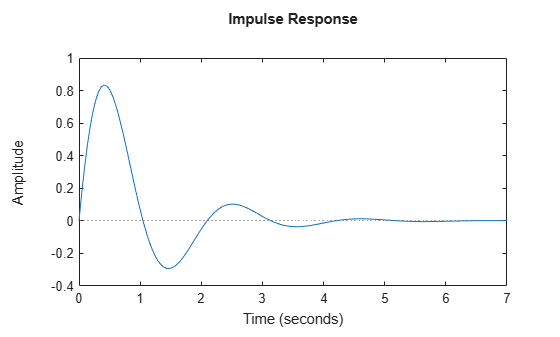The `impulse` plot automatically includes a dotted horizontal line indicating the steady-state response. In a MATLAB® figure window, you can right-click on the plot to view other impulse-response characteristics such as peak response and transient time.

Plot the impulse response of a discrete-time system. The system has a sample time of 0.2 s and is represented by the following state-space matrices.

```A = [1.6 -0.7; 1 0]; B = [0.5; 0]; C = [0.1 0.1]; D = 0;```

Create the state-space model and plot its impulse response.

```sys = ss(A,B,C,D,0.2); impulse(sys)```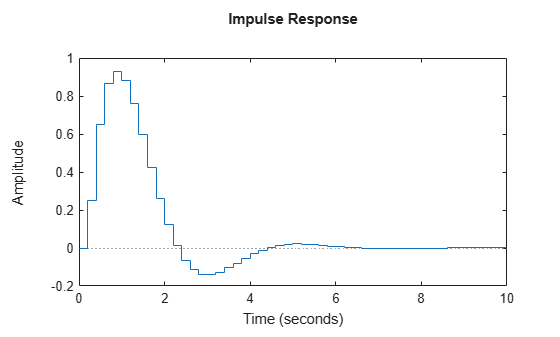The impulse response reflects the discretization of the model, as it shows the response as computed every 0.2 seconds.

Examine the impulse response of the following zero-pole-gain model.

`sys = zpk(-1,[-0.2+3j,-0.2-3j],1) * tf([1 1],[1 0.05]) `
```sys = (s+1)^2 ---------------------------- (s+0.05) (s^2 + 0.4s + 9.04) Continuous-time zero/pole/gain model. ```
`impulse(sys)`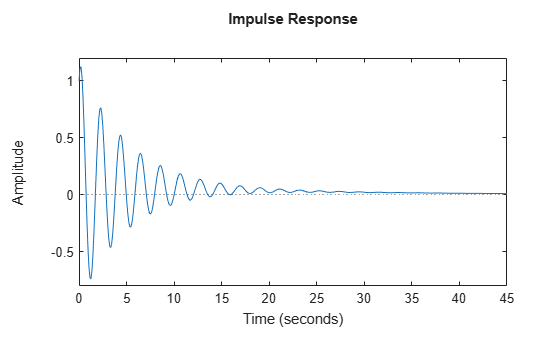By default, `impulse` chooses an end time that shows the steady state that the response is trending toward. To get a closer look at the transient response, limit the impulse plot to `t` = 20 s.

`impulse(sys,20)`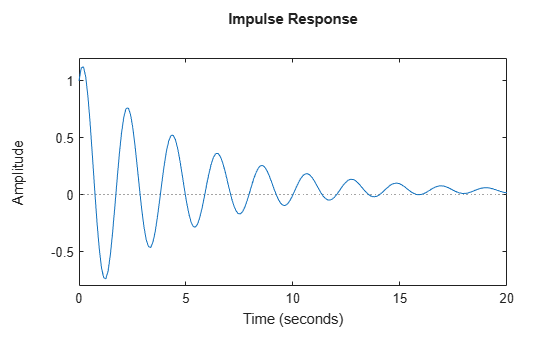Alternatively, you can specify the exact times at which you want to examine the impulse response, provided they are separated by a constant interval. For instance, examine the response from the end of the transient until the system reaches steady state.

```t = 20:0.2:120; impulse(sys,t)```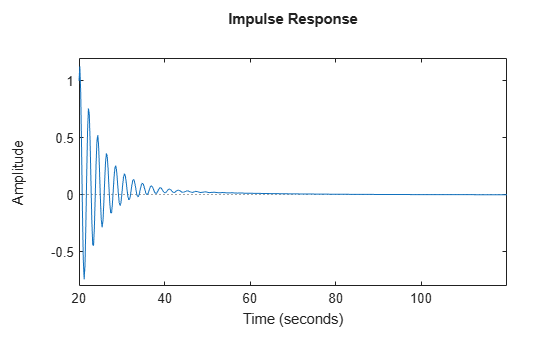Even though this plot begins at `t` = 20, `impulse` always applies the impulse input at `t` = 0.

Consider the following second-order state-space model:

`$\begin{array}{l}\left[\begin{array}{c}{\underset{}{\overset{˙}{x}}}_{1}\\ {\underset{}{\overset{˙}{x}}}_{2}\end{array}\right]=\left[\begin{array}{cc}-0.5572& -0.7814\\ 0.7814& 0\end{array}\right]\left[\begin{array}{c}{x}_{1}\\ {x}_{2}\end{array}\right]+\left[\begin{array}{cc}1& -1\\ 0& 2\end{array}\right]\left[\begin{array}{c}{u}_{1}\\ {u}_{2}\end{array}\right]\\ y=\left[\begin{array}{cc}1.9691& 6.4493\end{array}\right]\left[\begin{array}{c}{x}_{1}\\ {x}_{2}\end{array}\right]\end{array}$`

```A = [-0.5572,-0.7814;0.7814,0]; B = [1,-1;0,2]; C = [1.9691,6.4493]; sys = ss(A,B,C,0);```

This model has two inputs and one output, so it has two channels: from the first input to the output and from the second input to the output. Each channel has its own impulse response.

When you use `impulse`, it computes the responses of all channels.

`impulse(sys)`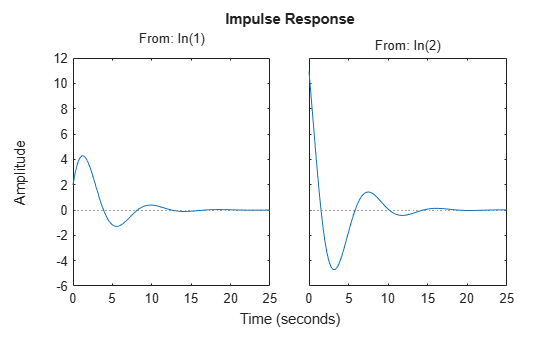The left plot shows the impulse response of the first input channel, and the right plot shows the impulse response of the second input channel. Whenever you use `impulse` to plot the responses of a MIMO model, it generates an array of plots representing all the I/O channels of the model. For instance, create a random state-space model with five states, three inputs, and two outputs, and plot its impulse response.

```sys = rss(5,2,3); impulse(sys)```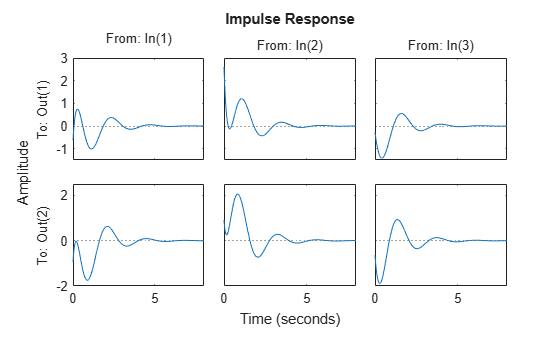In a MATLAB figure window, you can restrict the plot to a subset of channels by right-clicking on the plot and selecting I/O Selector.

`impulse` allows you to plot the responses of multiple dynamic systems on the same axis. For instance, compare the closed-loop response of a system with a PI controller and a PID controller. Create a transfer function of the system and tune the controllers.

```H = tf(4,[1 2 10]); C1 = pidtune(H,'PI'); C2 = pidtune(H,'PID');```

Form the closed-loop systems and plot their impulse responses.

```sys1 = feedback(H*C1,1); sys2 = feedback(H*C2,1); impulse(sys1,sys2) legend('PI','PID','Location','SouthEast')```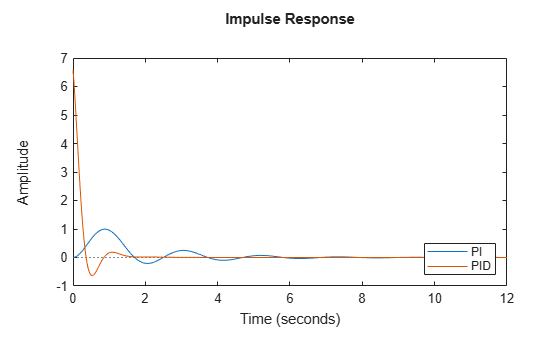By default, `impulse` chooses distinct colors for each system that you plot. You can specify colors and line styles using the `LineSpec` input argument.

``` impulse(sys1,'r--',sys2,'b') legend('PI','PID','Location','SouthEast')```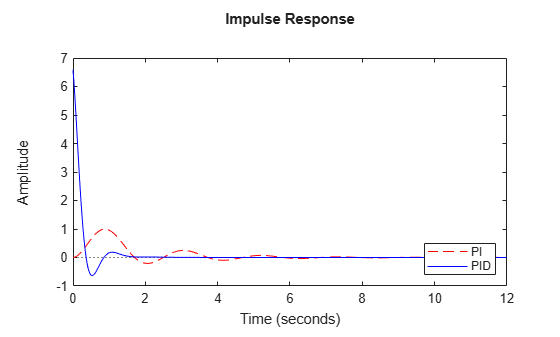The first `LineSpec` `'r--'` specifies a dashed red line for the response with the PI controller. The second `LineSpec` `'b'` specifies a solid blue line for the response with the PID controller. The legend reflects the specified colors and linestyles. For more plot customization options, use `impulseplot`.

The example Compare Impulse Response of Multiple Systems shows how to plot responses of several individual systems on a single axis. When you have multiple dynamic systems arranged in a model array, `impulse` plots all their responses at once.

Create a model array. For this example, use a one-dimensional array of second-order transfer functions having different natural frequencies. First, preallocate memory for the model array. The following command creates a 1-by-5 row of zero-gain SISO transfer functions. The first two dimensions represent the model outputs and inputs. The remaining dimensions are the array dimensions.

` sys = tf(zeros(1,1,1,5));`

Populate the array.

```w0 = 1.5:1:5.5; % natural frequencies zeta = 0.5; % damping constant for i = 1:length(w0) sys(:,:,1,i) = tf(w0(i)^2,[1 2*zeta*w0(i) w0(i)^2]); end```

(For more information about model arrays and how to create them, see Model Arrays (Control System Toolbox).) Plot the impulse responses of all models in the array.

`impulse(sys)`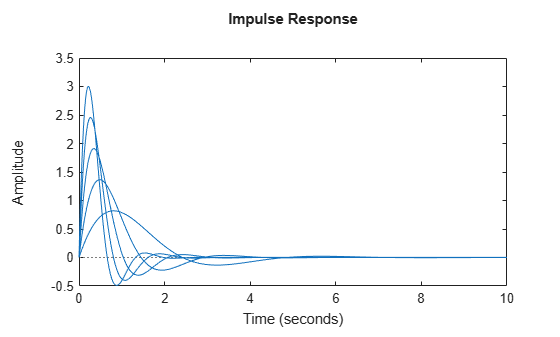`impulse` uses the same linestyle for the responses of all entries in the array. One way to distinguish among entries is to use the `SamplingGrid` property of dynamic system models to associate each entry in the array with the corresponding `w0` value.

`sys.SamplingGrid = struct('frequency',w0);`

Now, when you plot the responses in a MATLAB figure window, you can click a trace to see which frequency value it corresponds to.

When you give it an output argument, `impulse` returns an array of response data. For a SISO system, the response data is returned as a column vector of length equal to the number of time points at which the response is sampled. You can provide the vector t of time points, or allow `impulse` to select time points for you based on system dynamics. For instance, extract the impulse response of a SISO system at 101 time points between t = 0 and t = 5 s.

```sys = tf(4,[1 2 10]); t = 0:0.05:5; y = impulse(sys,t); size(y)```
```ans = 1×2 101 1 ```

For a MIMO system, the response data is returned in an array of dimensions N-by-Ny-by-Nu, where Ny and Nu are the number of outputs and inputs of the dynamic system. For instance, consider the following state-space model, representing a two-input, one-output system.

```A = [-0.5572,-0.7814;0.7814,0]; B = [1,-1;0,2]; C = [1.9691,6.4493]; sys = ss(A,B,C,0);```

Extract the impulse response of this system at 200 time points between t = 0 and t = 20 s.

```t = linspace(0,20,200); y = impulse(sys,t); size(y)```
```ans = 1×3 200 1 2 ```

`y(:,i,j)` is a column vector containing the impulse response from the jth input to the ith output at the times t. For instance, extract the impulse response from the second input to the output.

```y12 = y(:,1,2); plot(t,y12)```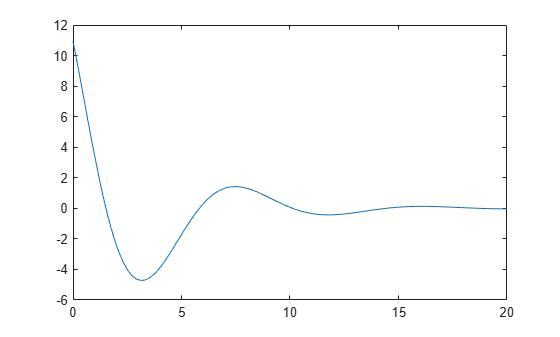Compare the impulse response of a parametric identified model to a non-parametric (empirical) model. Also view their 3 $\sigma$ confidence regions.

`load iddata1 z1`

Estimate a parametric model.

`sys1 = ssest(z1,4);`

Estimate a non-parametric model.

`sys2 = impulseest(z1);`

Plot the impulse responses for comparison.

```t = (0:0.1:10)'; [y1, ~, ~, ysd1] = impulse(sys1,t); [y2, ~, ~, ysd2] = impulse(sys2,t); plot(t, y1, 'b', t, y1+3*ysd1, 'b:', t, y1-3*ysd1, 'b:') hold on plot(t, y2, 'g', t, y2+3*ysd2, 'g:', t, y2-3*ysd2, 'g:')```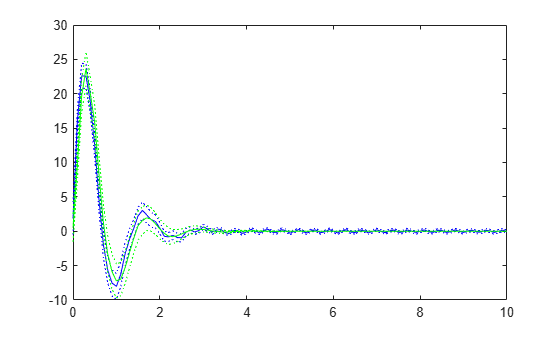Compute the impulse response of an identified time-series model.

A time-series model, also called a signal model, is one without measured input signals. The impulse plot of this model uses its (unmeasured) noise channel as the input channel to which the impulse signal is applied.

`load iddata9;`

Estimate a time-series model.

`sys = ar(z9, 4);`

`sys` is a model of the form `A y(t) = e(t)` , where `e(t)` represents the noise channel. For computation of impulse response, `e(t)` is treated as an input channel, and is named `e@y1`.

Plot the impulse response.

`impulse(sys)`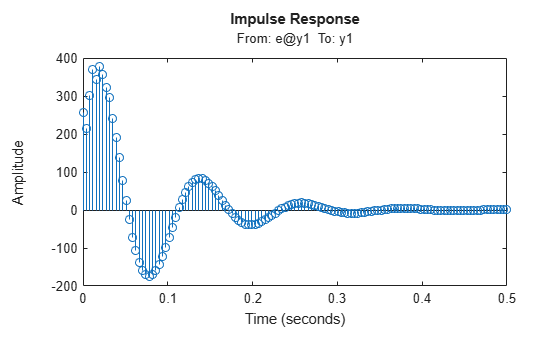Create a state-space model.

```A = [-0.8429,-0.2134;-0.5162,-1.2139]; B = [0.7254,0.7147;0,-0.2050]; C = [-0.1241,1.4090;1.4897,1.4172]; D = [0.6715,0.7172;-1.2075,0]; sys = ss(A,B,C,D);```

Create a default option set and use the dot notation to specify values.

```respOpt = RespConfig; respOpt.InputOffset = [-2,3]; respOpt.Amplitude = [2,-0.5]; respOpt.InitialState = [0.1,-0.1]; respOpt.Delay = 5;```

Compute the impulse response.

```t = 0:0.1:20; impulse(sys,t,respOpt)```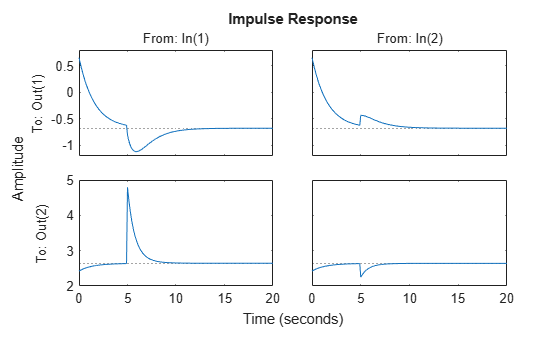This example shows how to simulate the impulse response of an LPV model. This example simulates the closed-loop response of a levitating ball model defined in `fcnMaglev.m` to a disturbance $\mathit{du}$.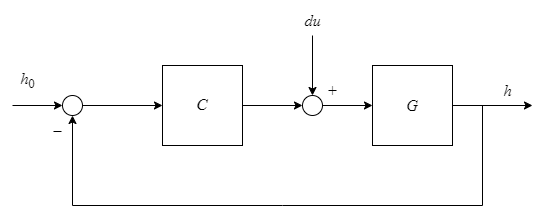You must set the reference to ${\mathit{h}}_{0}$ to properly initialize the system and maintain it around $\mathit{h}$ = ${\mathit{h}}_{0}$.

Create the model and discretize it.

```hmin = 0.05; hmax = 0.25; h0 = (hmin+hmax)/2; Ts = 0.01; Glpv = lpvss("h",@fcnMaglev,0,0,h0); Glpvd = c2d(Glpv,Ts,'tustin'); ```

Sample the LPV model for three height values and tune a PID controller.

```hpid = linspace(hmin,hmax,3); [Ga,Goffset] = sample(Glpvd,[],hpid); wc = 50; Ka = pidtune(Ga,'pidf',wc); Ka.Tf = 0.01;```

Create the gain-scheduled PID controller.

```Ka.SamplingGrid = struct('h',hpid); Koffset = struct('y',{Goffset.u}); Clpv = ssInterpolant(ss(Ka),Koffset);```

Create the closed-loop model.

```CL = feedback(Glpvd*[1,Clpv],1,2,1); CL.InputName = {'du';'href'}; CL.OutputName = 'h';```

Get steady-state current for $\mathit{h}$ = ${\mathit{h}}_{0}$ to size the disturbance

`[~,~,~,~,~,~,~,u0] = Glpv.DataFunction(0,h0);`

Response to impulse change in $\mathit{du}$ and ${\mathit{h}}_{0}$.

```t = 0:Ts:2; pFcn = @(k,x,u) x(1); Config = RespConfig('InputOffset',[0;h0],'Amplitude',0.2*[u0;h0]*Ts,'Delay',0.5,'InitialParameter',h0); impulse(CL,t,pFcn,Config) title('Impulse disturbance in current and impulse change in height')```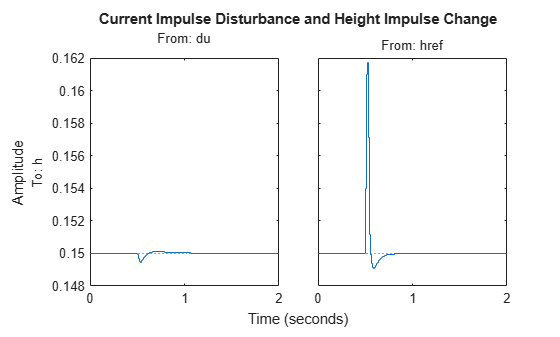## Input Arguments

collapse all

Dynamic system, specified as a SISO or MIMO dynamic system model or array of dynamic system models. Dynamic systems that you can use include:

• Continuous-time or discrete-time numeric LTI models, such as `tf`, `zpk`, or `ss` models.

• Generalized or uncertain LTI models such as `genss` or `uss` models. (Using uncertain models requires Robust Control Toolbox™ software.)

• For tunable control design blocks, the function evaluates the model at its current value for both plotting and returning response data.

• For uncertain control design blocks, the function plots the nominal value and random samples of the model. When you use output arguments, the function returns response data for the nominal model only.

• Sparse state-space models such as `sparss` and `mechss` models. You must specify final time `tFinal` for sparse state-space models.

• Identified LTI models, such as `idtf`, `idss`, or `idproc` models. For such models, the function can also plot confidence intervals and return standard deviations of the frequency response.

• Linear time-varying (`ltvss` (Control System Toolbox)) and linear parameter-varying (`lpvss` (Control System Toolbox)) models.

`impulse` does not support frequency-response data models such as `frd`, `genfrd`, or `idfrd` models.

If `sys` is an array of models, the function plots the responses of all models in the array on the same axes.

End time for impulse response, specified as a positive scalar value. `impulse` simulates the impulse response from ```t = 0``` to `t = ``tFinal`.

• For continuous-time systems, the function determines the step size and number of points automatically from system dynamics. Express `tFinal` in the system time units, specified in the `TimeUnit` property of `sys`.

• For discrete-time systems, the function uses the sample time of `sys` as the step size. Express `tFinal` in the system time units, specified in the `TimeUnit` property of `sys`.

• For discrete-time systems with unspecified sample time (```Ts = -1```), `impulse` interprets `tFinal` as the number of sampling periods to simulate.

Since R2023b

Time range for the impulse response, specified as a two-element vector of positive scalar values. `impulse` simulates the response from ```t = t0``` to `t = tFinal`.

If you have specified an impulse delay `td` using `RespConfig`, the function applies the impulse at ```t = t0+td```.

Time vector at which to compute the impulse response, specified as a vector of positive scalar values. Express `t` in the system time units, specified in the `TimeUnit` property of `sys`.

• For continuous-time models, specify `t` in the form `T0:dt:Tf`. To obtain the response at each time step, the function uses `dt` as the sample time of a discrete approximation to the continuous system. The simulation starts at `T0` and ends at `Tf`

• For discrete-time models, specify `t` in the form `T0:Ts:Tf`, where `Ts` is the sample time of `sys`.

Parameter trajectory of the LPV model, specified as a matrix or a function handle.

• For exogenous or explicit trajectories, specify `p` as a matrix with dimensions N-by-Np, where N is the number of time samples and Np is the number of parameters.

Thus, the row vector `p(i,:)` contains the parameter values at the ith time step.

• For endogenous or implicit trajectories, specify `p` as a function handle of the form p = F(k,x,u) that gives parameters as a function of time sample k, state x, and input u. `impulse` only supports this option for discrete-time LPV models.

This option is useful when you want to simulate quasi-LPV models.

Configuration of the applied impulse signal, specified as a `RespConfig` option set. By default, `impulse` applies an input at time `t = 0`. Use this input argument to change the response configuration such as specify delay or input offset. See Configure Options for Impulse Response (Control System Toolbox) for an example.

For `lpvss` (Control System Toolbox) and `ltvss` (Control System Toolbox) models with offsets (x0(t),u0(t)), you can use `RespConfig` to define the input relative to u0(t,p) and initialize the simulation with the state x0(t,p).

## Output Arguments

collapse all

Impulse response data, returned as an array.

• For SISO systems, `y` is a column vector of the same length as `t` (if provided) or `tOut` (if you do not provide `t`).

• For single-input, multi-output systems, `y` is a matrix with as many rows as time samples and as many columns as outputs. Thus, the jth column of `y`, or `y(:,j)`, contains the impulse response of from the input to the jth output.

• For MIMO systems, the impulse responses of each input channel are stacked up along the third dimension of `y`. The dimensions of `y` are then N-by-Ny-by-Nu, where:

• N is the number of time samples.

• Ny is the number of system outputs.

• Nu is the number of system inputs.

Thus, `y(:,i,j)` is a column vector containing the impulse response from the jth input to the ith output at the times specified in `t` or `tOut`.

Times at which impulse response is computed, returned as a vector. When you do not provide a specific vector `t` of times, `impulse` chooses this time vector based on the system dynamics. The times are expressed in the time units of `sys`.

State trajectories, returned as an array. When `sys` is a state-space model, `x` contains the evolution of the states of `sys` at each time in `t` or `tOut`. The dimensions of `x` are N-by-Nx-by-Nu, where:

• N is the number of time samples.

• Nx is the number of states.

• Nu is the number of system inputs.

Thus, the evolution of the states in response to an impulse injected at the kth input is given by the array `x(:,:,k)`. The row vector `x(i,:,k)` contains the state values at the ith time step.

Standard deviation of the impulse response of an identified model, returned as an array of the same dimensions as `y`. If `sys` does not contain parameter covariance information, then `ysd` is empty.

Parameter trajectories, returned as an array. When `sys` is a linear-parameter varying model, `pOut` contains the evolution of the parameters of `sys` at each time in `t` or `tOut`. The dimensions of `pOut` are N-by-Np-by-Nu, where:

• N is the number of time samples.

• Np is the number of parameters.

• Nu is the number of system inputs.

Thus, the evolution of the parameters in response to a signal injected at the kth input is given by the array `pOut(:,:,k)`. The row vector `pOut(i,:,k)` contains the parameter values at the ith time step.

## Limitations

• The impulse response of a continuous system with nonzero D matrix is infinite at t = 0. `impulse` ignores this discontinuity and returns the lower continuity value Cb at t = 0.

• The `impulse` command does not work on continuous-time models with internal delays. For such models, use `pade` (Control System Toolbox) to approximate the time delay before computing the impulse response.

• The `impulse` command does not support simulation along an implicit parameter trajectory for continuous-time LPV models.

## Algorithms

Continuous-time LTI models are first converted to state-space form. The impulse response of a single-input state-space model

`$\begin{array}{l}\stackrel{˙}{x}=Ax+bu\\ y=Cx\end{array}$`

is equivalent to the following unforced response with initial state b.

`$\begin{array}{cc}\stackrel{˙}{x}=Ax,& x\left(0\right)=b\\ y=Cx& \end{array}$`

To simulate this response, the system is discretized using zero-order hold on the inputs. The sample time is chosen automatically based on the system dynamics, except when a time vector `t = T0:dt:Tf` is supplied. Hence, `dt` is used as sample time.

## Version History

Introduced before R2006a

expand all